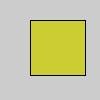Name

>> (right shift)

Examplesm = 8 >> 3   # In binary: 1000 to 1
print(m)   # Prints "1"
n = 256 >> 6 # In binary: 100000000 to 100
print(n)   # Prints "4"
o = 16 >> 3  # In binary: 10000 to 10
print(o)   # Prints "2"
p = 26 >> 1  # In binary: 11010 to 1101
print(p)   # Prints "13"# Using "right shift" as a faster technique than red(), green(), and blue()
argb = color(204, 204, 51, 255)
a = (argb >> 24) & 0xFF
r = (argb >> 16) & 0xFF  # Faster way of getting red(argb)
g = (argb >> 8) & 0xFF   # Faster way of getting green(argb)
b = argb & 0xFF          # Faster way of getting blue(argb)
fill(r, g, b, a)
rect(30, 20, 55, 55)
Description Shifts bits to the right. The number to the left of the operator is shifted the number of places specified by the number to the right. Each shift to the right halves the number, therefore each left shift divides the original number by 2. Use the right shift for fast divisions or to extract an individual number from a packed number. Right shifting only works with integers or numbers which automatically convert to an integer such at byte and char.

Bit shifting is helpful when using the color data type. A right shift can extract red, green, blue, and alpha values from a color. A left shift can be used to quickly reassemble a color value (more quickly than the color() function).
Syntax
value >> n
Parameters
value int: the value to shift int: the number of places to shift right
Related << (left shift)

Updated on Mon Sep 21 15:53:25 2020.

If you see any errors or have comments, please let us know.